# Rational numbers + natural numbers - math problems

#### Number of problems found: 16

• Closest natural numberFind the closest natural number to 4.456 to 44.56 and 445.6.
• What is 14What is the product of 0.97 and the next odd decimal number?
• FractionFraction ? write as fraction a/b, a, b is integers numerator/denominator.
• What is 11What is the quotient of Three-fifths and 1 Over 10?
• Cubic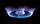Convert 660 m3 of natural gas volume to energy units kWh. Combustion of 1 cubic meter of natural gas is released energy 10.55 kWh.
• Year 2020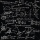The four-digit number divided by 2020 gives a result of 1, **. (Can not be in form 1,*0. ) Write all the options.
• Fraction to decimal infinite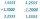Determine which digit is at 1000th place after the decimal point in the decimal expansion of the fraction 9/28.
• Tenths digit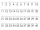For 10.932, which digit is in the tenths place?
• Homework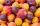In the crate are 18 plums, 27 apricot and 36 nuts. How many pieces of fruit left in the crate when Peter took 8 ninth: 1. nuts 2. apricots 3. fruit 4. drupe
• Cash back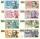After a trip 24 students have left more than 650 but less than 690 CZK (Kc). How much each student receives return when the amount is divisible by the number of pupils?
• Camp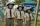In the camp are children. 1/2 went on a trip, 1/4 went to bathe and 38 children remained in the room. How many children are in camp?
• Blades1st blade 2,5 m, 2nd blade. .1.75 m. How many same long pieces of this two blades can be do the biggest? How long is one piece?
• Four pupilsFour pupils divided \$ 1485 so that the second received 50% less than the first, the third 1/2 less than a fourth, and fourth \$ 154 less than the first. How much money had each of them?
• My fatherMy father has a big farm. 6/8 of it were planted with mango trees, 1/2 of the remainder are guava trees and the rest 10 trees are santol trees. What is the number of all trees?
• DiophantusWe know little about this Greek mathematician from Alexandria, except that he lived around 3rd century A. D. Thanks to an admirer of his, who described his life through an algebraic riddle, we know at least something about his life. Diophantus's youth las
• BoatsThree-quarters of boats are white, 1/7 are blue and 9 are red. How many boats do we have?

Do you have an interesting mathematical word problem that you can't solve it? Submit a math problem, and we can try to solve it.

We will send a solution to your e-mail address. Solved examples are also published here. Please enter the e-mail correctly and check whether you don't have a full mailbox.

Please do not submit problems from current active competitions such as Mathematical Olympiad, correspondence seminars etc...
Rational numbers - math problems. Natural numbers - math problems.